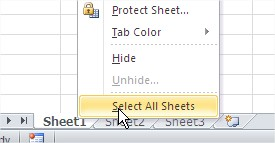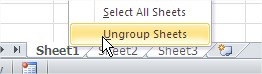#### How to Edit Multiple Excel Sheets at Once

In this lesson you can learn how to edit many worksheets at the same time.

Excel allows you to edit multiple sheets at the same time. This can be useful for tasks such as applying the same formula to multiple sheets or creating a consistent layout across multiple sheets.

## How to Select Multiple Sheets

Right-click on any tab and select the worksheet Select All Sheets.This will select all sheets.

When you are making changes to any sheet, these changes will also be present at all the selected sheets.

## How to Edit Multiple Sheets

To ungroup sheets, and then work on one, right-click at any tab and select Ungroup Sheets.## Using 3-D references

3-D references allow you to reference the same cell or range in multiple sheets. This means that any changes you make to the cell or range will be reflected in all sheets that reference it.

For example, if you have a formula in cell A1 of Sheet1 that calculates the total sales for all sheets, you can use a 3-D reference to reference the formula in Sheet2 and Sheet3. This way, if you change the formula in Sheet1, the changes will be reflected in Sheet2 and Sheet3 as well.

## Using VBA macros

VBA macros allow you to automate tasks in Excel. This means that you can write a macro to perform an operation on multiple sheets. This can be especially useful if you need to perform repetitive tasks.

For example, you could write a macro to copy the contents of a range in Sheet1 to the same range in Sheet2 and Sheet3. This would save you the time of having to manually copy the data to each sheet.

## Using Named Ranges

Named ranges are a powerful tool that can be used to make your Excel spreadsheets more efficient and easier to use. Named ranges allow you to give a name to a range of cells, which you can then refer to in formulas, macros, and other areas of your spreadsheet.

One of the most useful applications of named ranges is for editing multiple Excel sheets at once. By using named ranges, you can quickly and easily update data in multiple sheets without having to type the same formula or range of cells multiple times.

To use named ranges to edit multiple Excel sheets at once, you first need to create the named ranges. You can do this by selecting the range of cells that you want to name and then typing the name in the Name Box.

Once you have created the named ranges, you can use them in formulas and macros. For example, if you have a named range called TotalSales that contains the total sales for all sheets, you can use that named range in a formula to calculate the total sales for a specific sheet.

Here is an example of how you can use named ranges to edit multiple Excel sheets at once:

Suppose you have a workbook with three sheets named “Sheet1”, “Sheet2”, and “Sheet3”. Each sheet contains a range of cells that contains the total sales for that sheet. You can use named ranges to update the total sales for all sheets at once by using the following formula:

`=SUM(TotalSales)`

This formula will sum the values in the TotalSales named range for all sheets.

`=Copy(TotalSales, Sheet2!TotalSales)`